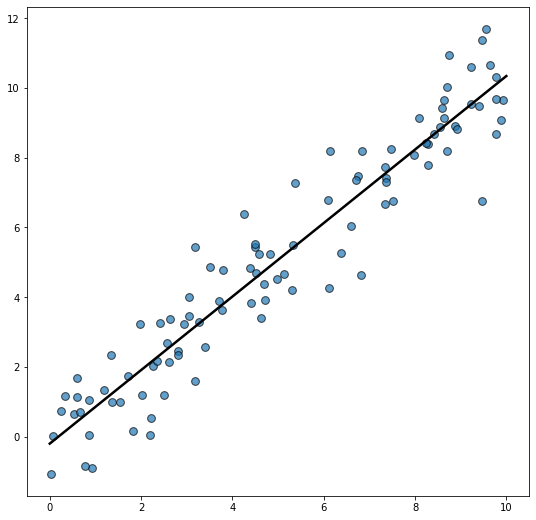# Scatterplot with regression line in Matplotlib

This guide shows how to plot a scatterplot with an overlayed regression line in Matplotlib. The linear regression fit is obtained with `numpy.polyfit(x, y)` where `x` and `y` are two one dimensional numpy arrays that contain the data shown in the scatterplot. The slope and intercept returned by this function are used to plot the regression line.

Let's get started by importing Matplotlib and Numpy

``````import matplotlib.pyplot as plt
import numpy as np``````

Now let's create a reproducible random number generator. This ensures the result is the same no matter how many times we generate the random data.

``rng = np.random.default_rng(1234)``

And finally, let's generate some random data, make the scatterplot, and add the regression line:

``````# Generate data
x = rng.uniform(0, 10, size=100)
y = x + rng.normal(size=100)

# Initialize layout
fig, ax = plt.subplots(figsize = (9, 9))

ax.scatter(x, y, s=60, alpha=0.7, edgecolors="k")

# Fit linear regression via least squares with numpy.polyfit
# It returns an slope (b) and intercept (a)
# deg=1 means linear fit (i.e. polynomial of degree 1)
b, a = np.polyfit(x, y, deg=1)

# Create sequence of 100 numbers from 0 to 100
xseq = np.linspace(0, 10, num=100)

# Plot regression line
ax.plot(xseq, a + b * xseq, color="k", lw=2.5);``````## Contact & Edit

👋 This document is a work by Yan Holtz. Any feedback is highly encouraged. You can fill an issue on Github, drop me a message onTwitter, or send an email pasting `yan.holtz.data` with `gmail.com`.